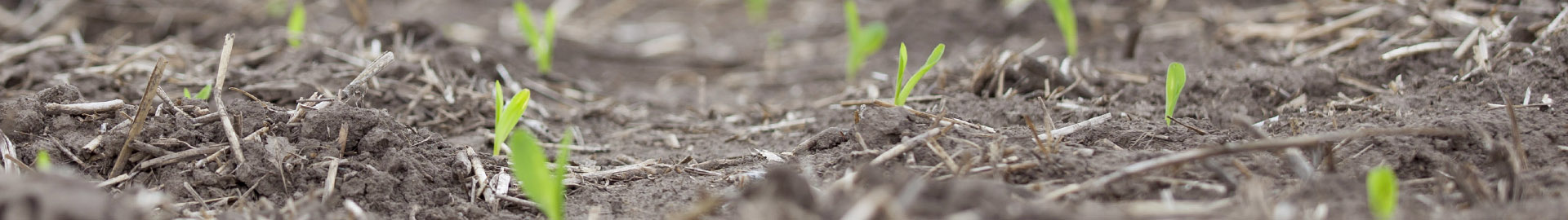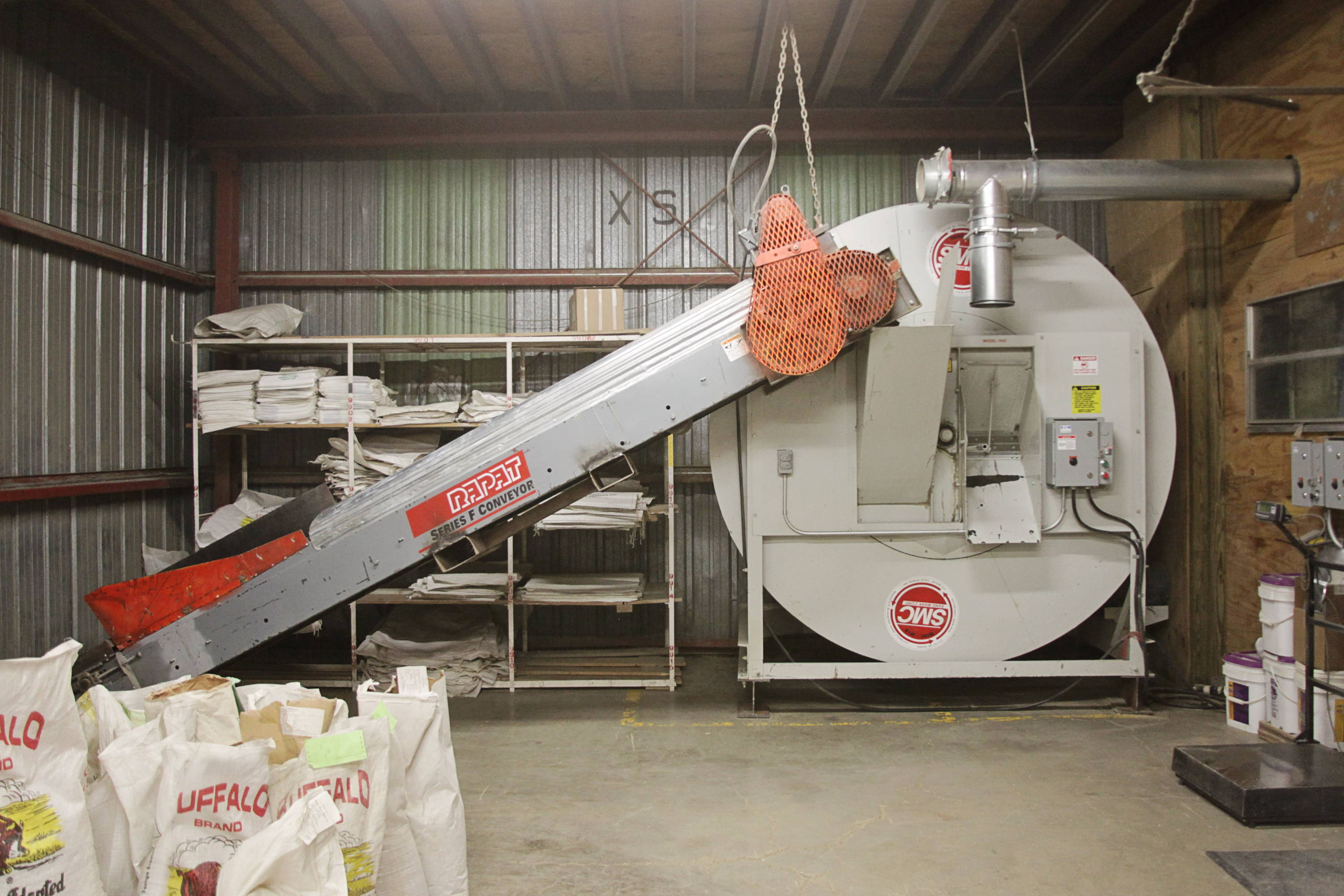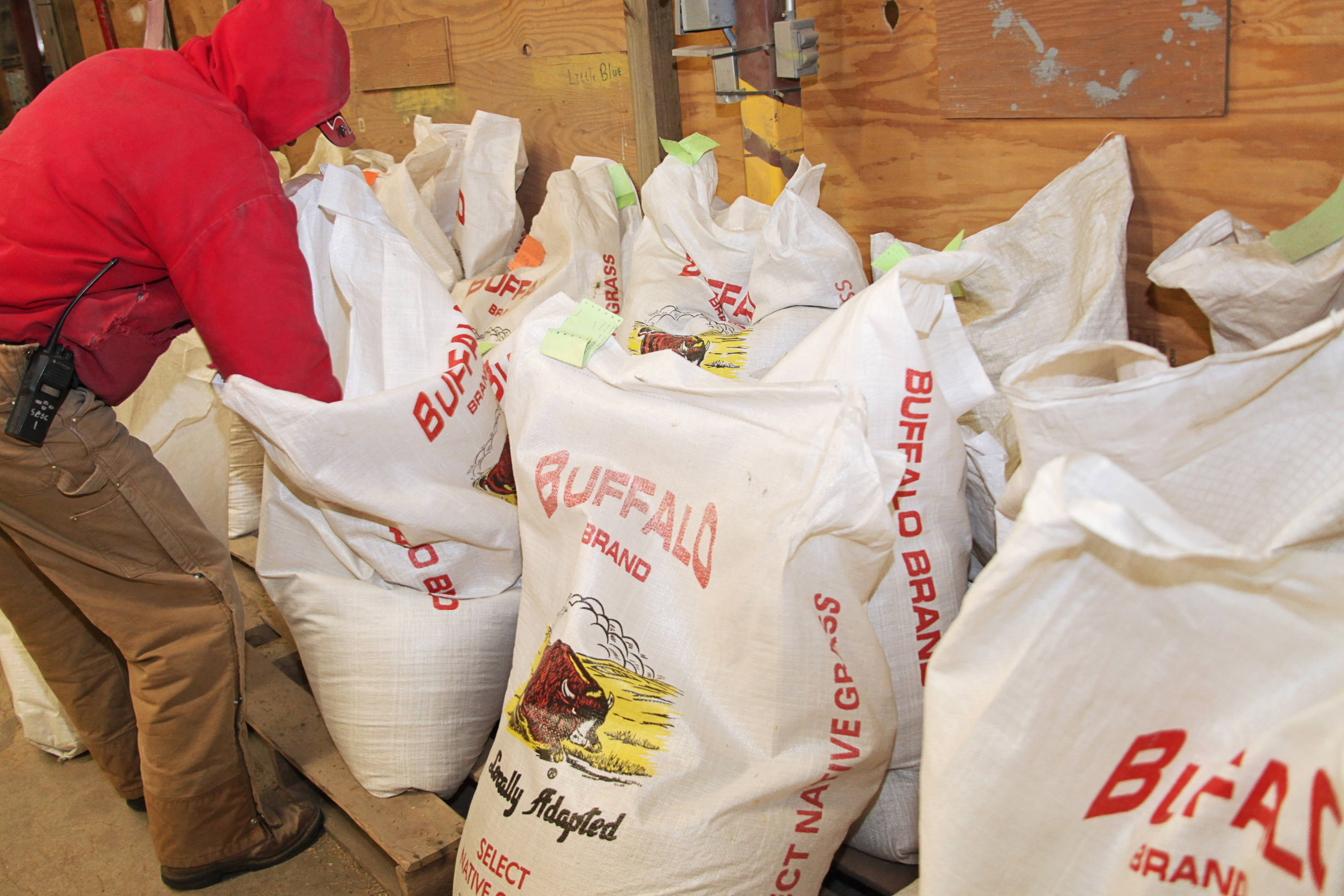# We Can Mix it Up!

## Let us take the quess work out of the equation.

Thanks!
This field is required
This field is required
This field is required# We Can Mix it Up!

## Let us take the quess work out of the equation.

Thanks!
This field is required
This field is required
This field is required# We Can Mix it Up!

## Let us take the quess work out of the equation.

Thanks!
This field is required
This field is required
This field is required